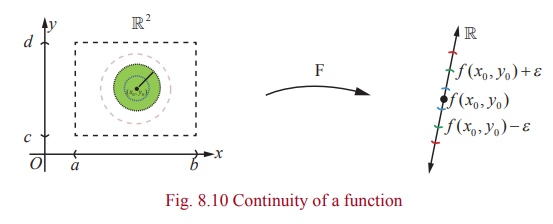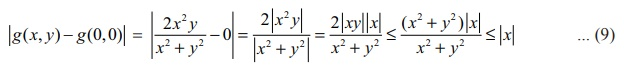Home | | Maths 12th Std | Limit and Continuity of Functions of Two Variables

# Limit and Continuity of Functions of Two Variables

Limit and Continuity of Functions of Two Variables: Definition 8.6 (Limit of a Function), Definition 8.7 (Continuity)

Limit and Continuity of Functions of Two Variables

### Definition 8.6 (Limit of a Function)

Suppose that A {( x , y) | a < x < b , c < y < d } ŌŖé ŌäØ2 , F : A ŌåÆ R. We say that F has a limit L at (u,v) if the following hold :

For every neighboourhood ( L ŌłÆ ╬Ą , L + ╬Ą ), ╬Ą > 0 , of L , there exists a ╬┤ ŌĆōneighbourhood B╬┤ ((u, v )) ŌŖé A of (u,v) such that ( x , y) Ōłł B╬┤ ((u, v )) \ {(u, v )}, ╬┤ > 0 ŌćÆ f (x) Ōłł(L ŌłÆ ╬Ą , L + ╬Ą ) .

We denote this by lim ( x , y ) ŌåÆ(u,v) F ( x, y ) = L if such a limit exists.When compared to the case of a function of single variable, for a function of two variables, there is a subtle depth in the limiting process. Here the values of F ( x, y) should approach the same value L , as ( x , y) approaches (u , v) along every possible path to (u , v) (including paths that are not straight lines). Fig.8.9 explains the limiting process.

All the rules for limits (limit theorems) for functions of one variable also hold true for functions of several variables.

Now, following the idea of continuity for functions of one variable, we define continuity of a function of two variables.

### Definition 8.7 (Continuity)

Suppose that A = {( x, y ) | a < x < b, c < y < d } ŌŖé ŌäØ2 , F : A ŌåÆ ŌäØ. We say that F is continuous at at (u , v) if the following hold :

(1) F is defined at (u , v)

(2) lim( x , y ) ŌåÆ(u,v) F ( x, y ) = L exists

(3) L = F (u,v).Remark

(1) In Fig. 8.10 taking L = F ( x0 , y0 ) will illustrate continuity at ( x0 , y0 ) .

(2) Continuity for f (x1 , x2 , , xn ) is also defined similarly as defined above.

Let us consider few examples as illustrations to understand continuity of functions of two variables.

Example 8.8

Let f (x , y) =(or) 3xŌłÆ5y+8 / x2+y2+1 for all ( x , y) Ōłł ŌäØ2. Show that f is continuous on ŌäØ2.

Solution

Let (a , b) Ōłł ŌäØ2 be an arbitrary point. We shall investigate continuity of f at (a , b).

That is, we shall check if all the three conditions for continuity hold for f at (a , b) .

To check first condition, note that f (a, b)is defined.Now we note that limx,yŌåÆ( a , b) f (x, y ) = L = f (a, b) . Hence f satisfies all the three conditions for continuity of f at (a , b) . Since (a , b) is an arbitrary point in ŌäØ2 , we conclude that f is continuous at every point of ŌäØ2.

Example 8.9

Consider f ( x, y)if ( x , y) ŌēĀ (0, 0) and f (0, 0) = 0 . Show that f is not continuous at (0, 0) and continuous at all other points of ŌäØ2 .

Solution

Note that f is defined for every ( x , y) Ōłł R2 . First let us check the continuity at ( a , b) ŌēĀ (0, 0) .

Let us say, just for instance, (a , b) = (2, 5) . Then f (2, 5) = 10/29 . Then, as in the above example, we  calculateit follows that f is continuous at (2, 5) .

Exactly by similar arguments we can show that f is continuous at every point ( a , b) ŌēĀ (0, 0) . Now let us check the continuity at (0, 0) . Note that f (0, 0) = 0 by definition. Next we want to find ifexists or not.

First let us check the limit along the straight lines y = mx , passing through (0, 0).So for different values of m , we get different values m / 1+ m2 and hence we conclude thatdoes not exist. Hence f cannot be continuous at (0, 0) .

Example 8.10

Consider g ( x , y) =if ( x , y) ŌēĀ (0, 0) and g(0, 0) = 0. Show that g is continuous on ŌäØ2.

Solution

Observe that the function g is defined for all ( x , y) Ōłł ŌäØ2 . It is easy to check, as in the above examples, that g is continuous at all point ( x , y) ŌēĀ (0, 0) . Next we shall check the continuity of g at (0, 0) . For that we see if g has a limit L at (0, 0) and if L = g(0, 0) = 0 . So we considerNote that in the final step above we have used 2|xy| Ōēż x2 + y2 (which follows by considering 0 Ōēż (x ŌłÆ y)2 ) for all x , y Ōłł ŌäØ. Note that ( x , y) ŌåÆ (0, 0) implies |x| ŌåÆ 0 . Then from (9) it follows that= 0 = g(0, 0) ; which proves that g is continuous at (0, 0) . So g is continuous at every point of ŌäØ2.

Tags : Mathematics , 12th Maths : UNIT 8 : Differentials and Partial Derivatives
Study Material, Lecturing Notes, Assignment, Reference, Wiki description explanation, brief detail
12th Maths : UNIT 8 : Differentials and Partial Derivatives : Limit and Continuity of Functions of Two Variables | Mathematics